# How to use the SUMIF and SUMIFS functions in formulas in Excel 2007 and Excel 2010 with examples

## Introduction

Hi and welcome to my latest hub on Excel. Today, I am going to look at how to use the SUMIF and SUMIFS functions in formulas in Excel.

• The SUMIF function allows you to sum (or add up) data based on a single criteria. In the example I will be using today, I want to sum all of sales of the different media types in my music shop regardless of artist (so I want total CD, LP and Cassette sales).

In other words using my example, Excel will SUM the contents of the cell IF the media type equals CD.

• The SUMIFS function allows you to sum data based on any number of criteria you wish. You can use up to 127 different criteria (that would be one heck of a long formula) if you so desire. Today, by way of an example, I will be using SUMIFS to add up the number of CDs, DVDs and LPs by each artist that I have sold.

The advantage of using SUMIF and also SUMIFS is that they are very easy to use. In addition, using the example of my music shop, I am constantly selling (hopefully!) new music; I can easily update my SUMIF and SUMIFS formulas by simply increasing the ranges the formula is adding up as my sales increase. In the figure below, you can see that my SUMIF formula has capacity for another 182 sales.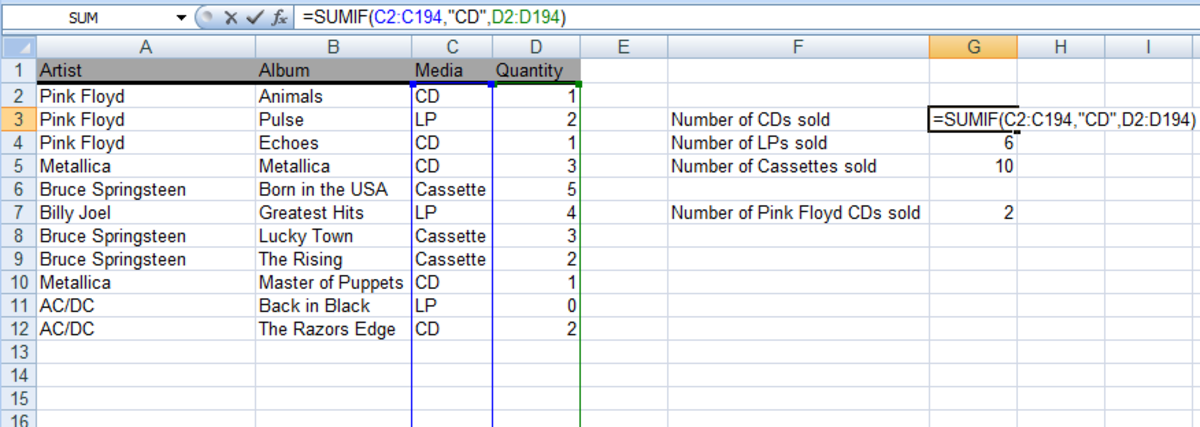An example of the SUMIF function used in a formula in Excel 2007 and Excel 2010.http://robbiecwilson.hubpages.com/

The IF function in Excel 2007 and Excel 2010 is very powerful and is made even more powerful when it is combined with other functions as is the case with SUMIF. I have a number of hubs covering different usages of the IF function including:

Using the COUNTIF and COUNTIFS function in formulas to count the number of times a particular item occurs in a list (I counted the number of pigs (COUNTIF) and the number of white pigs (COUNTIFS) I had on my farm by counting the number of times pig or white pig occurred on my list of the animals I have). To learn more about COUNTIF or COUNTIFS, click here:

http://robbiecwilson.hubpages.com/hub/Using-the-COUNTIF-and-COUNTIFS-formulas-and-the-Removing-Duplicates-button-in-Excel-2007

The IF function when combined with the logical functions AND, OR and NOT allows you to search for instances where two things happen together, when one or another occur (but not both) and when something doesn’t happen. This allows you to create powerful functions. I could search for CDs sales by Pink Floyd and AC/DC, or, Pink Floyd or AC/DC or, all CD sales not by AC/DC using these functions. This hub can be found here:

http://robbiecwilson.hubpages.com/hub/Using-the-IF-and-IFERROR-functions-as-well-as-the-logical-functions-AND-OR-and-NOT-in-Excel-2007-and-Excel-2010

Finally, I have used the IF function to convert the result of a formula into something I can use more easily. I used IF statements in my mortgage calculator to convert the item selected by the user of my spreadsheet into a number my formulas could use. That hub can also be found here:

http://robbiecwilson.hubpages.com/hub/Using-the-PMT-PPMT-IMPT-and-ABS-functions-to-create-a-Mortgage-Calculator-in-Excel-2007-and-Excel-2010

## Using the SUMIF function in formulas in Excel 2007 and Excel 2010

My music shop is selling albums on CD, LP and Cassette Tape. I would love to easily and quickly know how sales are doing on the different media types. All my sales are in recorded in Excel and I can use the SUMIF function in a formula to find out for example, how may CDs I have sold to date. You can see my list of sales below: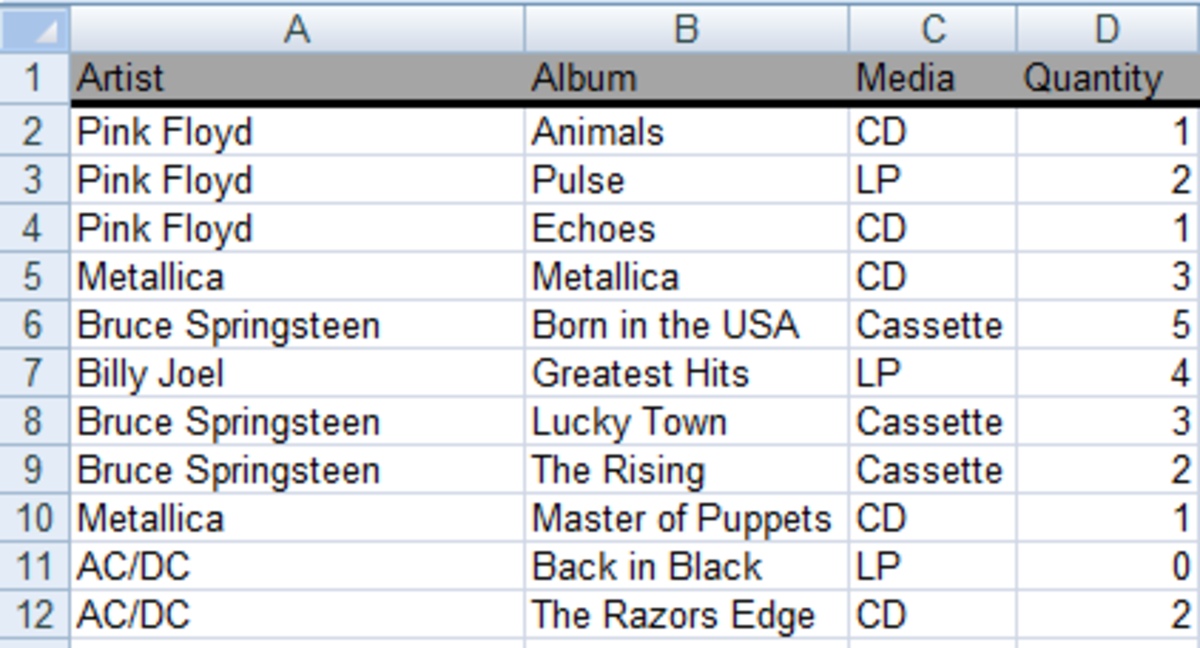List of sales to be used with the SUMIF function in Excel 2007 and Excel 2010.http://robbiecwilson.hubpages.com/

Now using SUMIF, I will calculate CDs sales across all the artists I stock. The SUMIF function consists of three parts:

1. The Criteria Range, which is the cells that contain whatever it is you want to sum (in my case CDs) which is C2 – C12
2. The Criteria, what it is you are going to sum (in this case “CD”)
3. The Sum Range, which is the cells that contain the cells you wish to sum (in my case sales quantities) which is cells D2 – D12

So to show this using SUMIF in a formula:

=SUMIF(C2:C12,"CD",D2:D12)

This is illustrated in the figure below, but in English I am looking in cells C2 – C12 and D2 – D12 and adding up all the rows in column D which contain CD in column C.

Scroll to Continue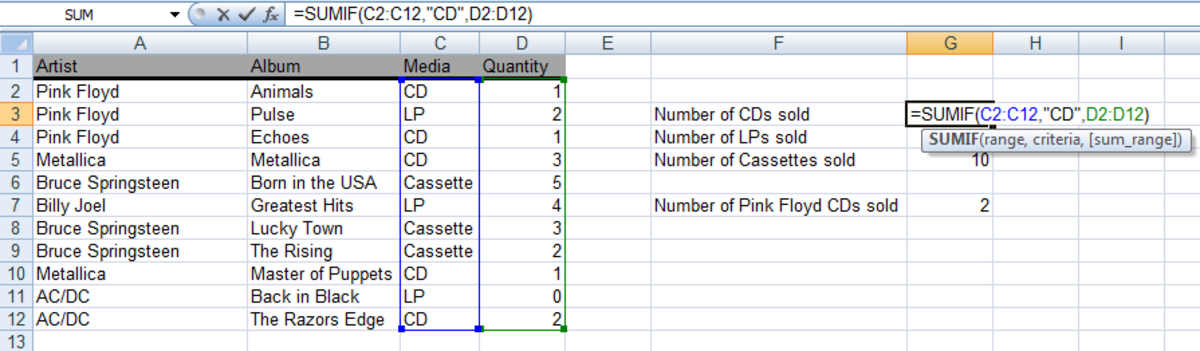Example of a SUMIF function used in a formula in Excel 2007 and Excel 2010.http://robbiecwilson.hubpages.com/

To add up all the sales of LPs and Cassette Tapes, I simply change the criteria (the part in the quotation marks ““) and leave the two ranges the same.

=SUMIF(C2:C12,"LP",D2:D12)

=SUMIF(C2:C12,"Cassette",D2:D12)

## Using the SUMIFS function in formulas in Excel 2007 and Excel 2010

The SUMIF formula is perfect if you only want to add up something based on a single criteria (in my example above, I wanted to add up all CD sales). But suppose I want to add up all sales of CDs by Pink Floyd; or if I wanted to add up all sales of the Pink Floyd album Animals on CD? I wouldn’t be able to use SUMIF as I have more than one criterion. In these cases I would have to use SUMIFS.

The syntax of the SUMIFS function is similar to SUMIF, with one important change.

Note: With SUMIFS, the Sum Range comes first rather than last. This is to avoid confusion with the multiple criteria that you (can) use.

After the Sum Range, next comes the Criteria Range and then the Criteria exactly as we did above with SUMIF.

Simply add any more additional criteria that you need to complete the formula. Now for an example:

I want to sum or add up, all the sales of Pink Floyd albums on CD. To do this:

• D2:D12 is my Sum range
• A2:A12 is my first criteria range
• “Pink Floyd” is my first criteria
• C2:C12 is my second criteria range
• “CD” is my second criteria

The formula becomes;

=SUMIFS(D2:D12,A2:A12,"Pink Floyd",C2:C12,"CD")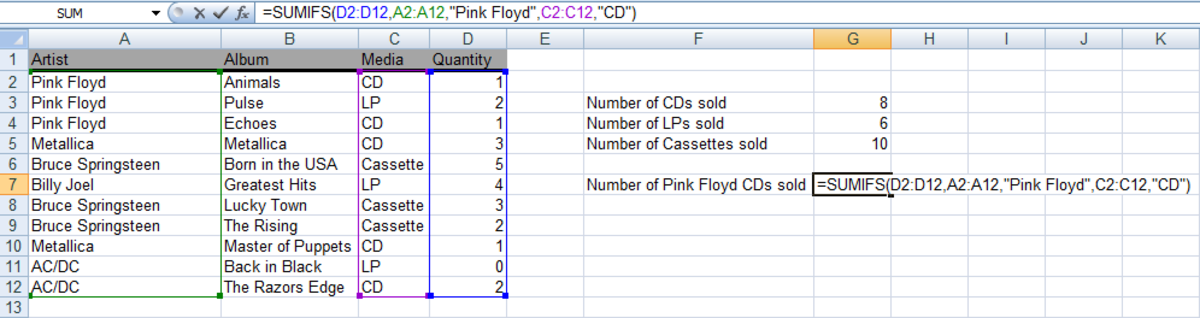Example of a SUMIFS function used in a formula in Excel 2007 and Excel 2010.http://robbiecwilson.hubpages.com/

If I want to add up all the Bruce Springsteen Cassettes I have sold, the formula is:

=SUMIFS(D2:D14,A2:A14,"Bruce Springsteen",C2:C14,"Cassette")

If I want to add up all the sales on CD of the Pink Floyd album Pulse, the formula would be:

=SUMIFS(D2:D12,A2:A12,"Pink Floyd",C2:C12,"CD",B2:B12,"Pulse")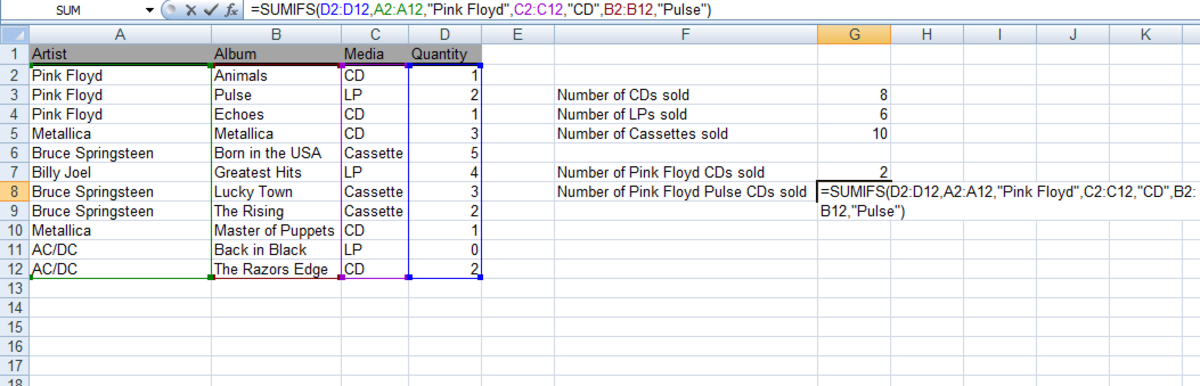A further example of a SUMIFS function used in a formula in Excel 2007 and Excel 2010http://robbiecwilson.hubpages.com/

Note: For both SUMIF and SUMIFS, you can use wild cards in the criteria. The ? can be used to represent one character and the * any number of cells. To use an example; Cassett? and Cass* could both be used to represent Cassette in my example above and would also still provide the correct results.

Note: If you actually want to use a ? or a * as an actual character and not a wild card, put a tilde ~ in front of it, to advise Excel to treat it as a character. So for example, if I wanted to use Who? in a SUMIF formula, I would use:

=SUMIF(C2:C12,"Who~?",D2:D12)

## Conclusion

The IF function in Excel 2007 and Excel 2010 is a very powerful and versatile function which can be used in formulas in Excel in a wide variety of ways. In today’s hub, I looked at the SUMIF and SUMIFS functions and how you can use them in formulas.

SUMIF sums (or adds up) data based on a single criteria. In my example, I added up all the sales of CDs in my music store.

SUMIFS sums data based on more than one criterion. So I added up all the sales of Pink Floyd on CD, as well as the sales of specific albums on CD.

Finally, we went through how to use wild cards correctly in SUMIF and SUMIFS.

Many thanks for reading; I hope that you found this hub both informative and useful. Please feel free to leave any comments you may have below.

Robbie C Wilson (author) on March 10, 2015:

Hi TTGReviews, thanks so much for your kind comment, I am so glad that you found my hub useful and informative.

TTGReviews on January 27, 2015:

I knew about SUMIF, but not about SUMIFS. This will be very helpful to know in the future.

Robbie C Wilson (author) on February 15, 2014:

Hi Daniel,

Thanks so much for your kind comments. Excel is such a massive and complicated application, I love to learn new things and then write about them. I am so glad that you found my article useful.

Daniel Wyvern from Athens, Georgia on February 07, 2014:

SUMIF is very powerful and I've used it many times over the years. I am always surprised at the number of people who were not even aware of it. But I have to admit that I was not aware of SUMIFS. Thanks for the knowledge.